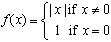#Interactive Real Analysis

Next | Previous | Glossary | Map

## 7.1. Riemann Integral

### Examples 7.1.6(b):

Why is, in general, an upper (or lower) sum not a special case of a Riemann sum ? Find a condition for a function f so that the upper and lower sums are actually special cases of Riemann sums.
The reason is simple: a Riemann sum requires points where the function is defined, while an upper/lower sum involves an sup/inf which may not correspond to points in the range of the function.

What this means is best illustrated via an example. Take the functionNow take the simple partition consisting of the interval [-1, 1]. Since f(x) > 0 for all x (which is not visible in the above applet) we must have that any Riemann sum R(f, P) must give a value strictly bigger than 0. The lower sum with respect to this partition, on the other hand, is truly zero (which again is not visible in the above applet (why?)). Therefore this particular lower sum can not be a Riemann sum.

So to find a condition that ensures that upper/lower sums are special cases of Riemann sums we must ensure that the sup/inf that appears in the definition of upper/lower sum is a max or min, respectively. In the topology chapter we have shown that a continuous function over a closed, bounded interval must have a max and a min.

Therefore, if f is continuous over the interval [a, b] then the upper and the lower sum are both special cases of a Riemann sum.

Next | Previous | Glossary | Map